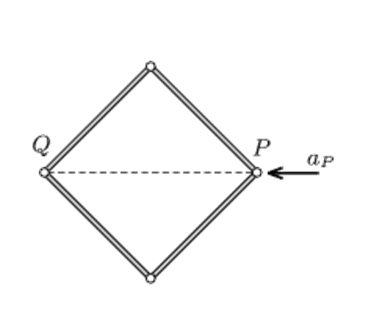# Acceleration of hingesFour similar rods of uniform density are connected with frictionless hinges. This frame is placed on a horizontal smooth tabletop, such that its shape is a square. Vertex P is acted upon by a horizontal force in the direction of the diagonal, and due to this force it begins to move at an acceleration of $a_P$.

If the initial acceleration of the opposite vertex Q is $a_Q=ka_P$ then find k.

k is positive if Q moves away from P and k is negative if Q moves closer to P

×